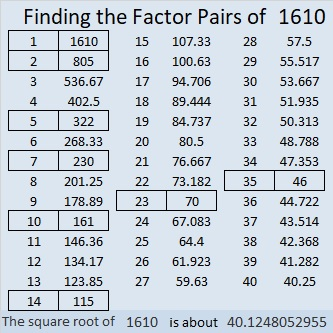# 1610 Four-Leaf Clovers

Contents

### Today’s Puzzle:

Sometimes four-leaf clovers are associated with Saint Patrick’s Day. Four-leaf clovers are supposed to be lucky, but you might not feel so lucky as you work on solving this puzzle. I assure you, there is a logical way to proceed on each step!Print the puzzles or type the solution in this excel file: 14 Factors 1604-1612.

### Factors of 1610:

• 1610 is a composite number.
• Prime factorization: 1610 = 2 × 5 × 7 × 23.
• 1610 has no exponents greater than 1 in its prime factorization, so √1610 cannot be simplified.
• The exponents in the prime factorization are 1, 1, 1, and 1. Adding one to each exponent and multiplying we get (1 + 1)(1 + 1)(1 + 1)(1 + 1) = 2 × 2 × 2 × 2 = 16. Therefore 1610 has exactly 16 factors.
• The factors of 1610 are outlined with their factor pair partners in the graphic below.### More about the Number 1610:

1610 is the hypotenuse of a Pythagorean triple:
966-1288-1610, which is (3-4-5) times 322.

1610 is not the sum of two squares or the difference of two squares. 1610 is a leg in some Pythagorean triples because
2(805)(1) = 1610,
2(161)(5) = 1610,
2(115)(7) = 1610, and
2(35)(23) = 1610.

You can calculate those Pythagorean triples by letting a be the first number in parenthesis for each of those equations, and b be the second number in parenthesis. Then substitute those values in the three expressions below, and you will have some Pythagorean triples!
2(a)(b), a² – b², a² + b².

This site uses Akismet to reduce spam. Learn how your comment data is processed.